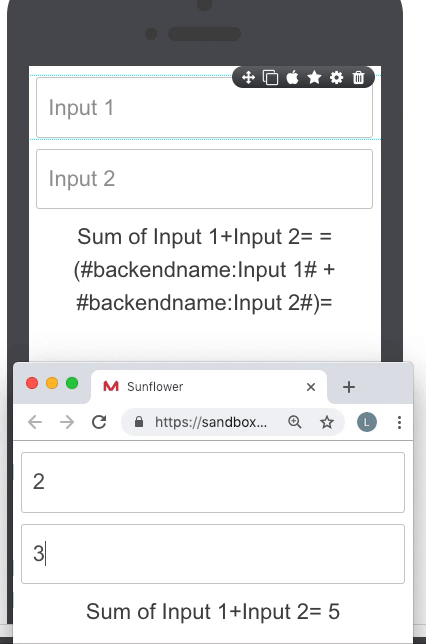# Use MathJS formulas

Formulas are used to perform mathematical operations within app. Mobsted platform supports all arithmenical functions and all MathJS formulas.

Use Hashtags to access data from any part of platform and build math expressions with it.

Example:

`=(  #hashtag1# + #hashtag2# )=`

OR

`  =( 3 + #hashtag2# )=`

Use case:

In the example below we can see that the sum of two fields is dynamically updated when we enter the new value to the one of the inputs.### AVAILABLE FORMULAS

 Action Example SUM / Multiply =( #hashtag1# + #hashtag2# )= / =( 5 * 2 )= Subtract / Divide =( #hashtag1# - #hashtag2# )= / =( 6 / 2 )= Modulus =( #hashtag1# % #hashtag2# )= Exponentiation =( #hashtag1# - #hashtag2# )= ^ =( #hashtag1# / #hashtag2# )= Square root =( sqrt(#hashtag1#) )= Factorial =( #hashtag1#! )=

You can check the set up of some of the above functions on screen - 4 Math expressions & operations in Demo App in your profile.